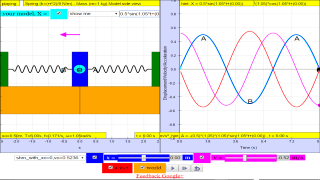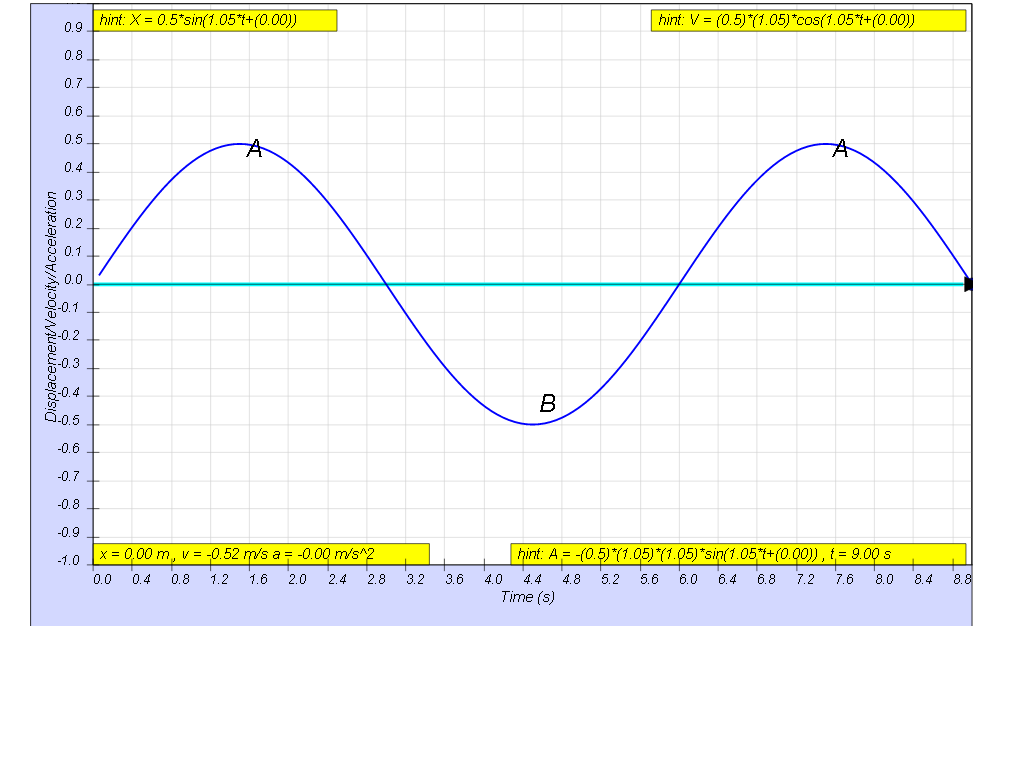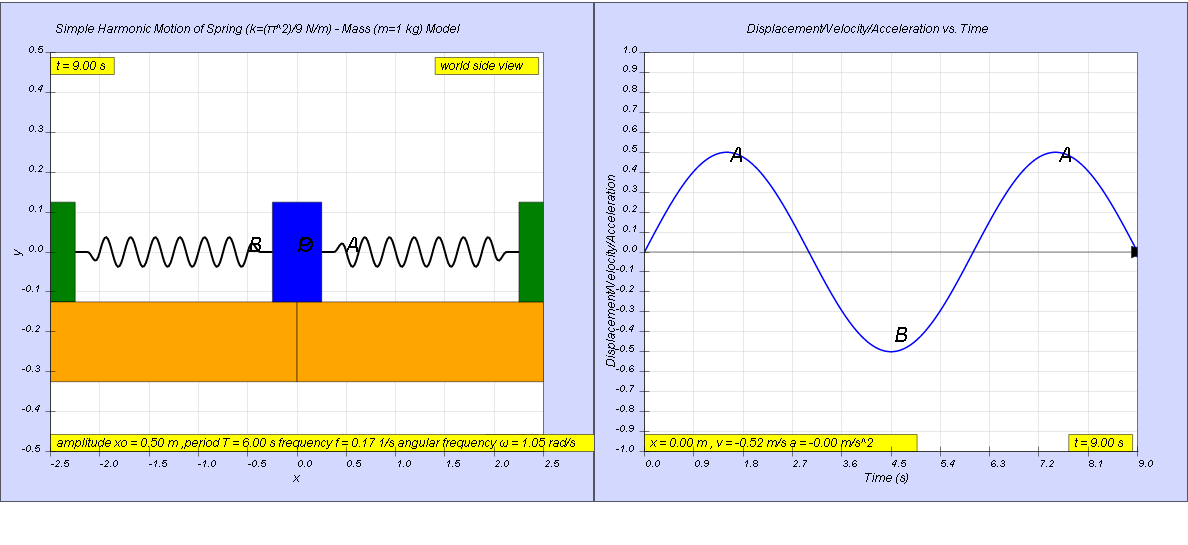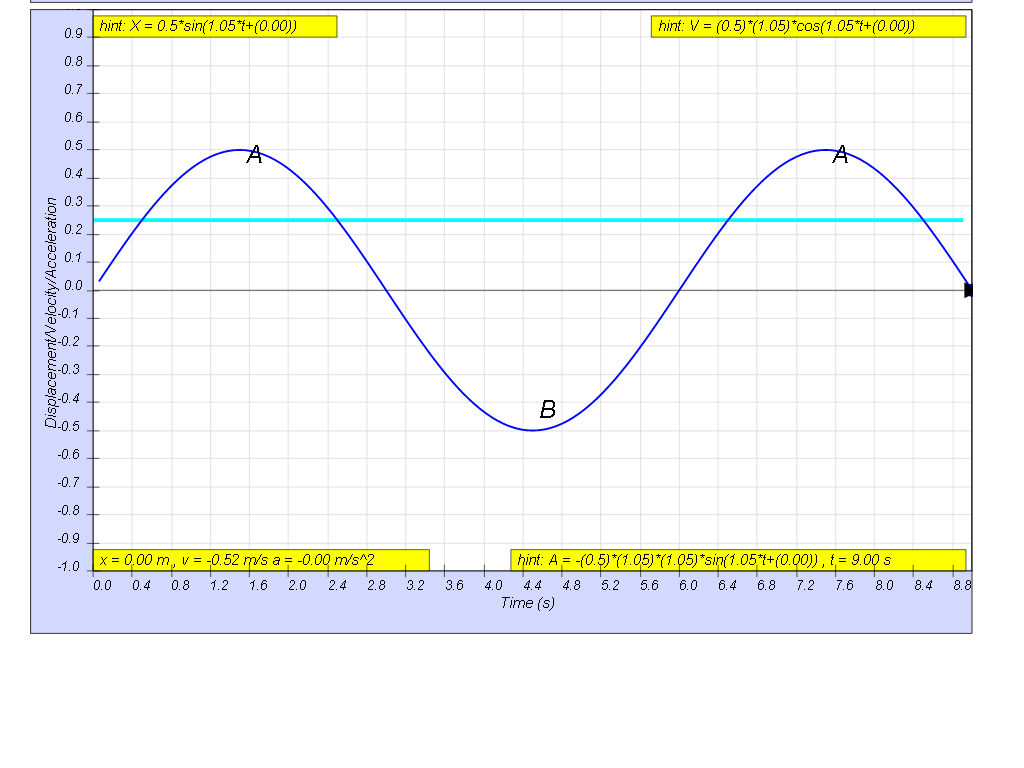## 10.2.7 Example Spring k=(π^2)/9 N/m, mass m=1 kg model versus Time for t = 1.5 Period JavaScript HTML5 Applet Simulation ModelSHM13

### 1.2.7 Example

A body moves in a simple harmonic motion and the following graph gives the variation of its displacement x with time t.a)    Write an equation to represent the given simple harmonic motion.
b)    Find the time duration in the first cycle when the body is located above a displacement of 0.25 m.

[ x = xo sin (1.05 t) , Δt = 1.9 s ]

## The hint can be found in the model### 1.2.7.1 Solution:

a) x0 = 0.5 m

$\mathrm{since \omega }=\genfrac{}{}{0.1ex}{}{2\pi }{T}$ $\mathrm{, \omega }=\genfrac{}{}{0.1ex}{}{2\pi }{6.0}$

ω = 1.05
x = x0sin(ω t) = 0.5 sin(1.05t)

b) At x = 0.25 m,

substituting back into the equation,

0.25 = 0.5 sin(1.05t)

solving for t gives

0.5 = sin(1.05t)


solving of the ranging of time, t

1.05t = 0.524 and π - 0.524

t1 = 0.5 , t2 = 2.4 s

looking at the graphical form of the solution of the 2 times, t1 and t2.

giving the range to be t2 - t1 = 2.4 - 1.5 = 1.9 s### Translations

Code Language Translator Run### Software Requirements

SoftwareRequirements

 Android iOS Windows MacOS with best with Chrome Chrome Chrome Chrome support full-screen? Yes. Chrome/Opera No. Firefox/ Samsung Internet Not yet Yes Yes cannot work on some mobile browser that don't understand JavaScript such as..... cannot work on Internet Explorer 9 and below

### CreditsThis email address is being protected from spambots. You need JavaScript enabled to view it.

### end faq

http://iwant2study.org/lookangejss/02_newtonianmechanics_8oscillations/ejss_model_SHM13/SHM13_Simulation.xhtml